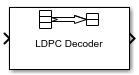# LDPC Decoder

Decode binary low-density parity-check (LDPC) code

• Library:
• Communications Toolbox / Error Detection and Correction / Block

•## Description

The LDPC Decoder block uses the belief propagation algorithm to decode a binary LDPC code, which is input to the block as the soft-decision output (log-likelihood ratio of received bits) from demodulation. The block decodes generic binary LDPC codes where no patterns in the parity-check matrix are assumed. For more information, see Belief Propagation Decoding.

The input and output are discrete-time signals. The ratio of the output sample time to the input sample time is:

• N/K when only the information-part of the codeword is decoded

• 1 when the entire codeword is decoded

N is the length of the received signal and must be in the range (0, 231). K is the length of the uncoded message and must be less than N.

This icon shows all ports, including optional ports, for the LDPC Decoder block.## Ports

### Input

expand all

Log-likelihood ratios, specified as an N-by-1 column vector containing the soft-decision output from demodulation. N is the number of bits in the LDPC codeword before modulation. Each element is the log-likelihood ratio for a received bit and the value is more likely to be `0` if the log-likelihood ratio is positive. The first K elements correspond to the information-part of the input message.

Data Types: `double`

### Output

expand all

Decoded data, returned as a column vector. The Decision type parameter specifies whether the block outputs hard decisions or soft decisions (log-likelihood ratios).

• If the Output format parameter is set to `Information part`, the output includes only the information-part of the received codeword.

• If the Output format parameter is set to `Whole codeword`, the output includes the whole log-likelihood ratio vector.

Data Types: `double` | `Boolean`

Number of executed decoding iterations, returned as a positive integer.

#### Dependencies

To enable this port, select the Output number of iterations executed parameter.

Data Types: `double`

Final parity checks after decoding the input LDPC code, returned as an (N-K)-by-1 column vector. N is the number of bits in the LDPC codeword before modulation. K is the length of the uncoded message.

#### Dependencies

To enable this port, select the Output final parity checks parameter.

## Parameters

expand all

Parity-check matrix, specified as a sparse (NK)-by-N binary-valued matrix. N is the length of the received signal and must be in the range (0, 231). K is the length of the uncoded message and must be less than N. The last (NK) columns in the parity-check matrix must be an invertible matrix in the Galois field of order 2, `gf`(2).

You can also specify the parity-check matrix as a two-column nonsparse index matrix, `I`, that defines the row and column indices of the `1`s in the parity-check matrix such that `sparse(I(:,1),I(:,2),1)`.

This parameter accepts numeric data types. When you set this parameter to a sparse binary matrix, this parameter also accepts the `Boolean` data type.

The default value uses the `dvbs2ldpc` function to configure a sparse parity-check matrix for half-rate LDPC coding, as specified in the DVB-S.2 standard.

Example: `dvbs2ldpc(R,'indices')` configures the index matrix for the DVB-S.2 standard, where `R` is the code rate, and `'indices'` specifies the output format of `dvbs2ldpc` as a two-column double-precision matrix that defines the row and column indices of the `1`s in the parity-check matrix.

Data Types: `double` | `Boolean`

Output value format, specified as one of these values:

• `Information part` — The block outputs a K-by-1 column vector containing only the information-part of the received log-likelihood ratio vector. K is the length of the uncoded message.

• `Whole codeword` — The block outputs an N-by-1 column vector containing the whole log-likelihood ratio vector. N is the length of the received signal.

N and K must align with the dimension of the (NK)-by-K parity-check matrix.

Decision method used for decoding, specified as one of these values:

• `Hard decision` — The block outputs decoded data of data type `double` or `boolean`. Specify this data type using the Output data type parameter.

• `Soft decision` — The block outputs log-likelihood ratios of data type `double`.

Output value data type, specified as `double` or `boolean`.

#### Dependencies

To enable this parameter, set the Decision type parameter to `Hard decision`.

Maximum number of decoding iterations, specified as a positive integer.

Select this parameter to terminate decoding after all parity checks are satisfied. If not all parity checks are satisfied, decoding terminates after the number of iterations specified by the Number of iterations parameter.

Select this parameter to enable the Iter output port.

Select this parameter to enable the ParChk output port.

## Block Characteristics

 Data Types `Boolean` | `double` Multidimensional Signals `no` Variable-Size Signals `no`

## Algorithms

expand all

This block performs LDPC decoding using the belief propagation algorithm, also known as a message-passing algorithm.

 Gallager, Robert G. Low-Density Parity-Check Codes. Cambridge, MA: MIT Press, 1963.